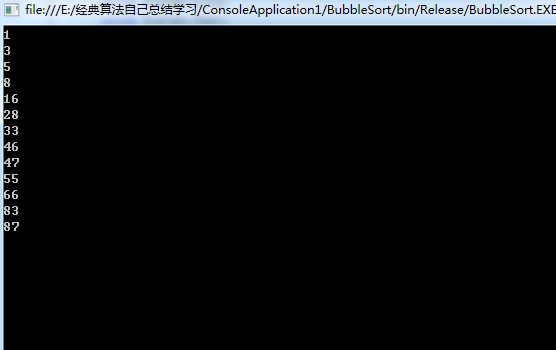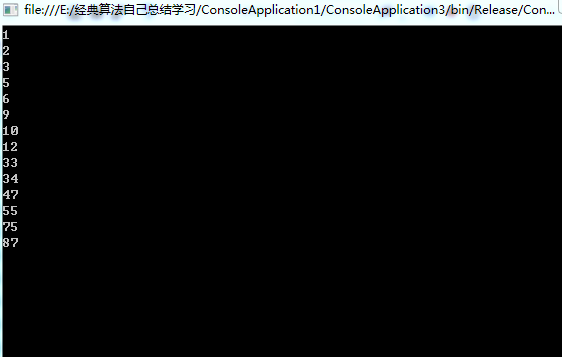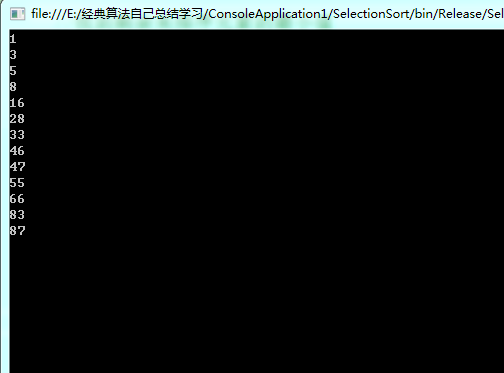# 排序算法的研究总结

2018/03/06 17:30

1, 5, 3, 83, 46, 55, 16, 87, 28, 66, 8, 33, 47

1.源代码

using System;
using System.Collections.Generic;
using System.Linq;
using System.Text;

namespace BubbleSort
{
class Program
{
static void Main(string[] args)
{
//定义要排序的数组
int[] iArrary = new int[] { 1, 5, 3, 83, 46, 55, 16, 87, 28, 66, 8, 33, 47 };

Program pg = new Program();

//给数组排序
pg.Sort(iArrary);

//排序后循环输出数组
foreach (int item in iArrary)
{
Console.WriteLine(item);

}
//防止控制台一闪而过

}

public void Sort(IList<int> data)
{
//从第一个数(i = 0)开始，循环整个数组。
for (int i = 0; i < data.Count; i++)
{
//从最后一个数开始，到第(i+1)个数结束，依次比较相邻的两个数，
//如果前一个数大于后一个数，就交换他们两个
//这样，第一个循环下来，第一个数是最小的数
//第二个循环下来，第二个数是第二小的数。。。依次类推
for (int j = data.Count-1; j > i; j--)
{
if (data[j - 1] > data[j])
{
int temp;
temp = data[j - 1];
data[j - 1] = data[j];
data[j] = temp;

}

}
}
}
}
}

2 .运行结果#### 2.算法描述

• <1>.从第一个元素开始，该元素可以认为已经被排序；
• <2>.取出下一个元素，在已经排序的元素序列中从后向前扫描；
• <3>.如果该元素（已排序）大于新元素，将该元素移到下一位置；
• <4>.重复步骤3，直到找到已排序的元素小于或者等于新元素的位置；
• <5>.将新元素插入到该位置后；
• <6>.重复步骤2~5。

3.源代码

using System;
using System.Collections.Generic;
using System.Linq;
using System.Text;

namespace ConsoleApplication3
{
class Program
{
static void Main(string[] args)
{
int[] iArrary = new int[] { 1, 5, 3, 6, 10, 55, 9, 2, 87, 12, 34, 75, 33, 47 };

Program pg = new Program();

pg.InsrtSort(iArrary);

foreach (int item in iArrary)
{
Console.WriteLine(item);

}

}

//插入排序
public void InsrtSort(IList<int> data)
{
// int[] iArrary = new int[] { 1, 5, 3, 6, 10, 55, 9, 2, 87, 12, 34, 75, 33, 47 };
int temp;

for (int i = 1; i < data.Count; i++)
{
temp = data[i];//要与已排序数组比较的新元素

//从0到j，代表的是已排序的数
for (int j = i - 1; j >= 0; j--)
{

if (data[j] > temp)//新元素小于该元素(已排序)，将该元素移到下一位置
{
data[j + 1] = data[j];
//data[j] = temp;
if (j == 0)//因为取不到 j==0 的值，故在此做特殊处理
{
data = temp;//只有当第二个数小于第一个数的时候，才会执行该处代码
}
}
else//否则，将新元素移到下一位置
{
data[j + 1] = temp;
break;//跳出里面的循环

}

}
}

}

}
}

4.运行结果1.思路

using System;
using System.Collections.Generic;
using System.Linq;
using System.Text;

namespace SelectionSort
{
class Program
{
static void Main(string[] args)
{

int[] iArrary = new int[] { 1, 5, 3, 83, 46, 55, 16, 87, 28, 66, 8, 33, 47 };

Program pg = new Program();

pg.Sort(iArrary);

foreach (int item in iArrary)
{
Console.WriteLine(item);

}

}
public void Sort(IList<int> data)
{
// int[] iArrary = new int[] { 1, 5, 3, 6, 10, 55, 9, 2, 87, 12, 34, 75, 33, 47 };
for (int i = 0; i < data.Count - 1; i++)
{
//在未排序序列中，总是默认为第一个值，为最小值；min 与temp都是过来跑龙套的
int min = i;//最小值的序号
int temp = data[i];//最小值

//找到剩余未排序元素的最小值
for (int j = i+1; j < data.Count; j++)
{
if (data[j] < temp)//剩余未排序元素中，存在某元素数小于最小值
{
min = j;
temp = data[j];//将该元素的值认为是最小值
}

}

if (min != i)//
{
int t = data[min];
data[min] = data[i];
data[i] = t;//将找到的最小值放入已排序序列的末尾

}

}

}

}
}

3.运行结果### 作者的其它热门文章

0
0 收藏

0 评论
0 收藏
0